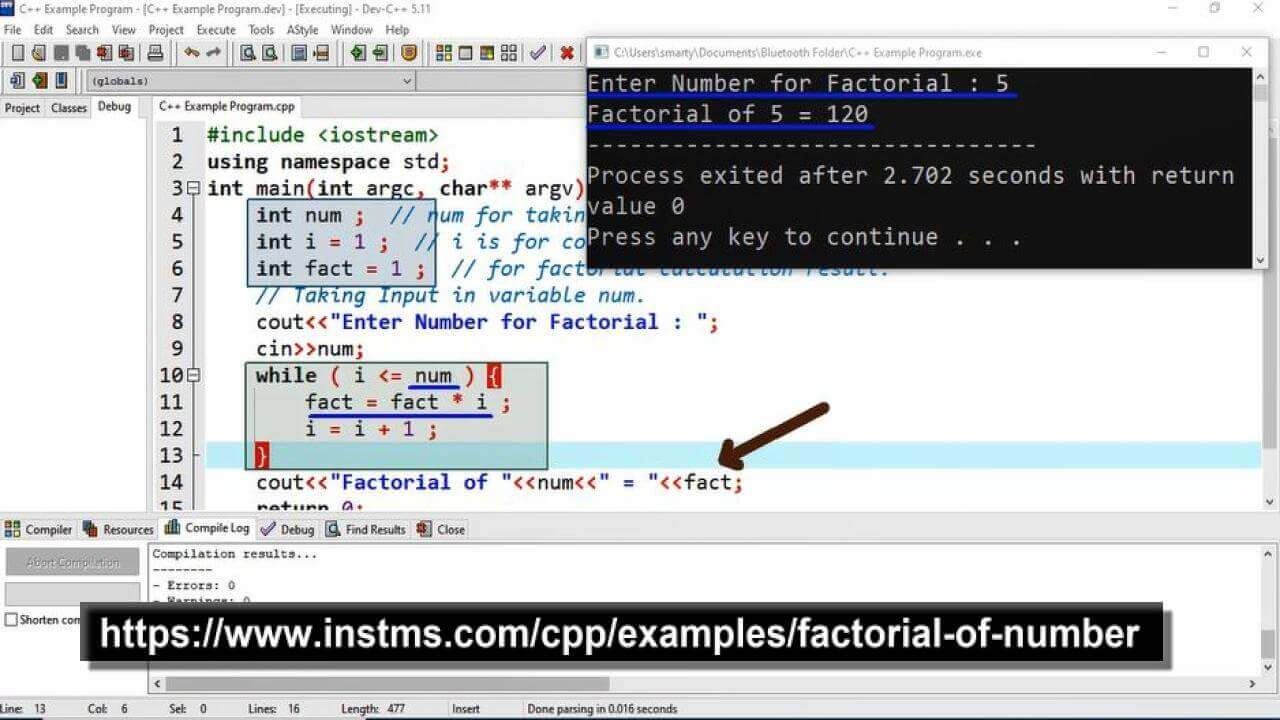# Factorial Of Number using CPP

## Source Code

#include <iostream>

using namespace std;

int main(int argc, char** argv) {
int num ;  // num for taking input from user.
int i = 1 ;  // i is for counter variable.
int fact = 1 ;  // for factorial calculation result.

// Taking Input in variable num.
cout<<"Enter Number for Factorial : ";
cin>>num;

while ( i <= num ) {
fact = fact * i ;
i = i + 1 ;
}

cout<<"Factorial of "<<num<<" = "<<fact;
return 0;
}

## Output## Working:

In this program example we take one variable for taking input from the user and other two variable are used for factorial calculation. These all variables are of same data type. Here is the list of variable along their use in this program example.

 Sr. Variable Name Data Type Description 1 num int This variable is declared to take input from user. 2 i int This is counter variable to track no of iterations. 3 fact int fact variable is used to stroe the result of multiplication. As result of last iteration this variable will have calculated factorial.

Here fact variable is initialized by 1, because it will be used to store the result of multiplication. So that we store multiplication identity in fact variable at the time of declaration. We set data type of fact as int but a common practice is long int used for its data type. Because this variable have to hold result of multiplication so that it is good practice to set it's data type ling int other than int. The other variable i is initialized by 1, because it will be used as counter variable.

In while loop we set condition as i <= num beacuse the mathematcial formula for calculation of factorial is.

num! = 1 x 2 x 3 ......... x (num-1) x num

So that we have to go from 1 to num in loop. We may use other than while loop such as for loop or do while loop. In last line of the program we print factorial using cout statement.